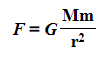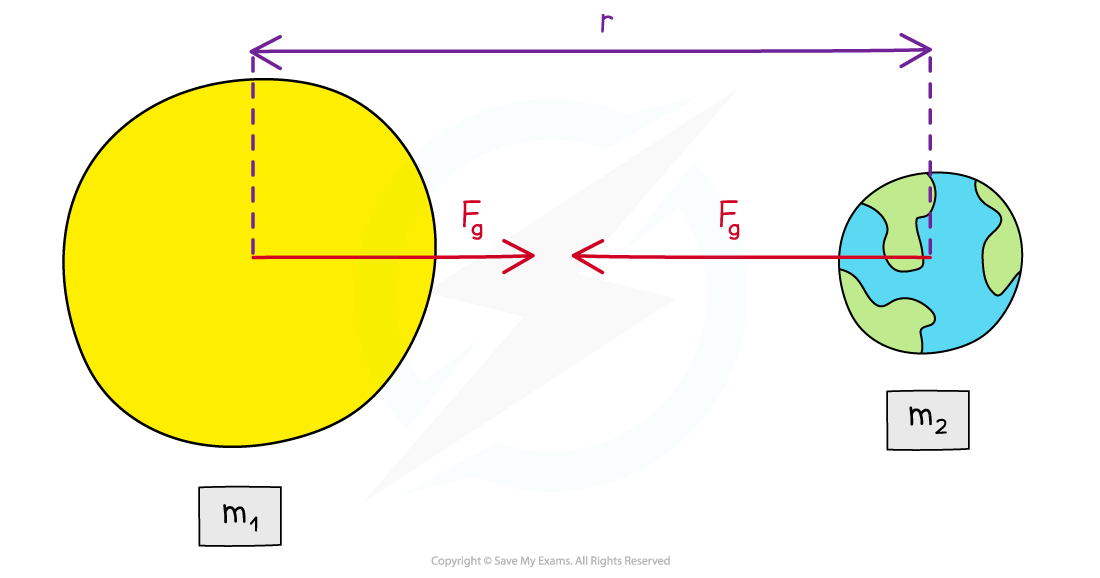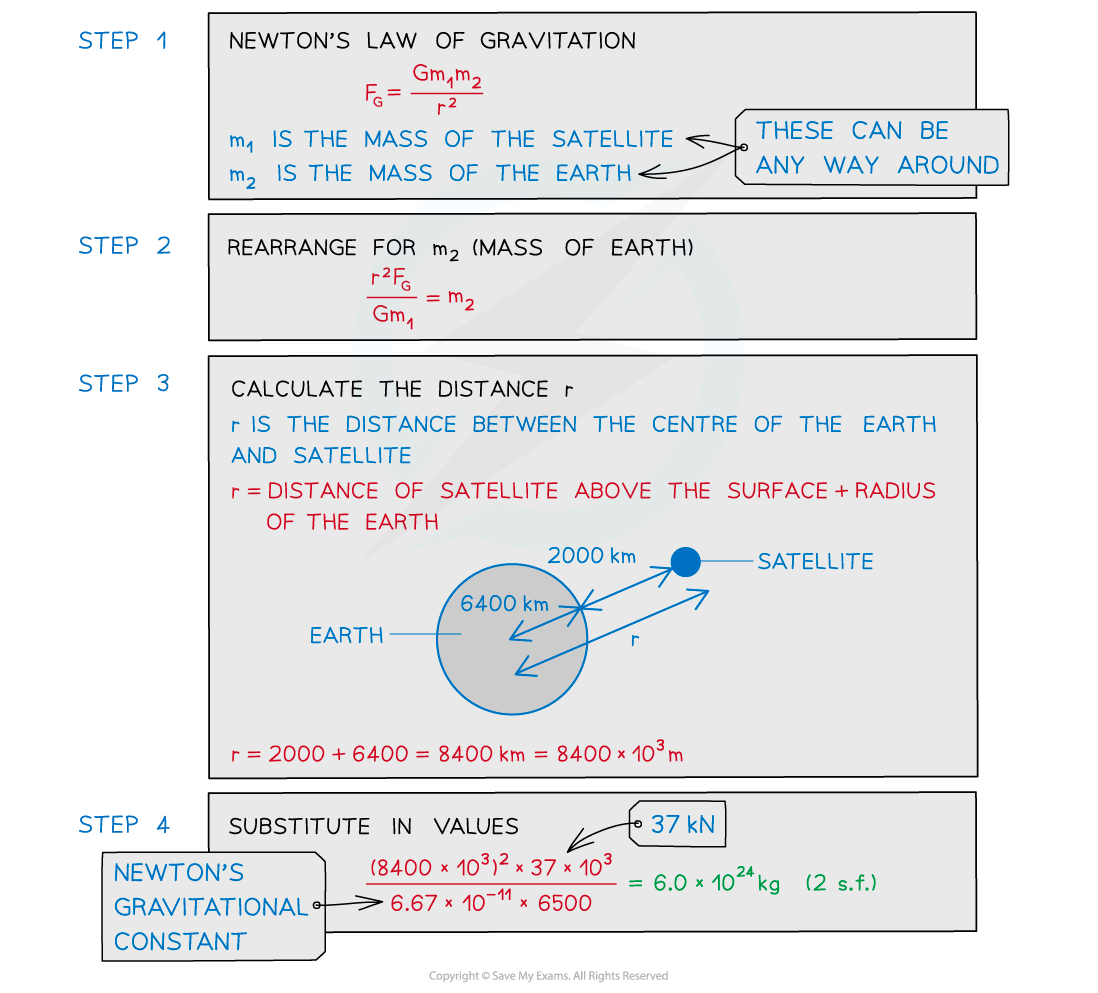# IB DP Physics: HL复习笔记6.2.1 Newton’s Law of Gravitation

### Newton's Law of Gravitation

• The gravitational force between two bodies outside a uniform field, e.g. between the Earth and the Sun, is defined by Newton’s Law of Gravitation
• Recall that the mass of a uniform sphere can be considered to be a point mass at its centre
• Newton’s Law of Gravitation states that:

The gravitational force between two point masses is proportional to the product of the masses and inversely proportional to the square of their separation

• In equation form, this can be written as:The gravitational force between two masses outside a uniform field is defined by Newton’s Law of Gravitation

• Where:
• F= gravitational force between two masses (N)
• G = Newton's Gravitational Constant
• m and = two points masses (kg) (These are sometimes labelled m1 and m2)
• r = distance between the centre of the two masses (m)
• Although planets are not point masses, their separation is much larger than their radius
• Therefore, Newton’s law of gravitation applies to planets orbiting the Sun
• The 1/r2 relation is called the ‘inverse square law’
• This means that when a mass is twice as far away from another, its force due to gravity reduces by (½)2 = ¼

#### Worked Example

A satellite with mass 6500 kg is orbiting the Earth at 2000 km above the Earth's surface. The gravitational force between them is 37 kN.

Calculate the mass of the Earth. (Radius of the Earth = 6400 km)#### Exam Tip

A common mistake in exams is to forget to add together the distance from the surface of the planet and its radius to obtain the value of r. The distance r is measured from the centre of the mass, which is from the centre of the planet.